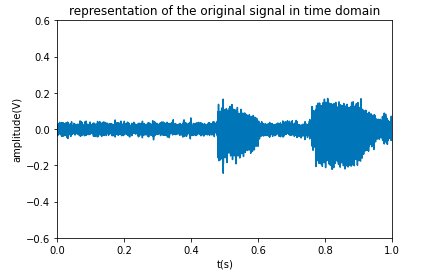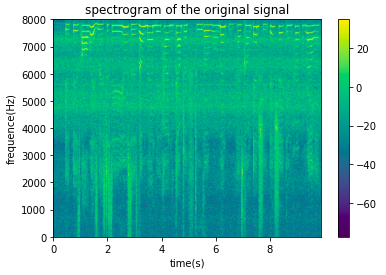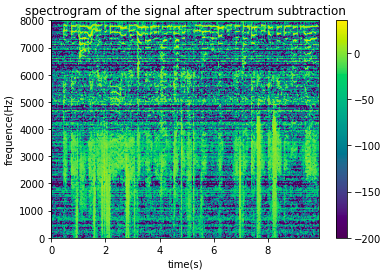# 使用谱减法去除音频底噪

Python音频信号处理 1.短时傅里叶变换及其逆变换

# Part 2 ：使用谱减法降低或消除信号的底噪

# 短时傅立叶变换
def TFCT(trame, Fe, Nfft,fenetre,Nwin,Nhop):
L = round((len(trame) - len(fenetre))/Nhop)+1
M = Nfft
xmat = np.zeros((M,L))
print('xmat',xmat.shape)
print(Nwin+Nhop)
for j in range(L):
xmat[:,j] = np.fft.fft(trame[j*Nhop:Nwin+Nhop*j]*window,Nfft)
x_temporel = np.linspace(0,(1/Fe)*len(trame),len(trame))
x_frequentiel = np.linspace(0, Fe,Nfft)

return xmat,x_temporel,x_frequentiel

# 短时傅立叶变换逆变换
def ITFD(xmat,Fe,Nfft,Nwin,Nhop):
window = np.hamming(Nwin)
Te = 1/Fe
yvect = np.zeros(Nfft + (xmat.shape-1)*Nhop)
t_vecteur = np.arange(0,Te*len(yvect),Te)
index = 0
K = 0
L = xmat.shape
yl = np.zeros((Nfft,L))
for j in range(L):
yl[:,j] = np.fft.ifft(xmat[:,j])
for k in range(L):
yvect[Nhop*k:Nfft+Nhop*k] += yl[:,k]
for n in range(Nwin-1):
K +=  window[n]
K /= Nhop
yvect /=K
print(yvect.shape)
return t_vecteur, yvect


# 读取初始信号 mix.wav
Fe, mix = wavfile.read('mix.wav')
mix = mix/2**15
Te = 1 / Fe
xtemp = np.arange(0,Te*len(mix),Te)
plt.figure()
plt.plot(xtemp,mix)
plt.axis([0,1,-0.6,0.6])
plt.xlabel('t(s)')
plt.ylabel('amplitude(V)')
plt.title('representation of the original signal in time domain')
display(Audio(mix,rate=Fe))



Fe为原始信号的采样频率，mix为复信号值。
Te = 1/Fe = 6.25e-5Nfft = 1024
Nwin = 1024
Nhop = 512
window = np.hamming(Nwin)
xmat_sound,tvect,fvect = TFCT(mix,Fe,Nfft,window,Nwin,Nhop)


xmat_sound就是我们保存短时傅立叶变换值的矩阵，该矩阵的每一行代表一个在0至采样频率范围内的频率，单位为Hz，每一列对应该段被窗函数截取的信号的FFT快速傅里叶变换值。

module_tf_xmat = abs(xmat_sound)
plt.figure()
xlim = int(module_tf_xmat.shape/2)
ylim = int(module_tf_xmat.shape/2)
plt.imshow(20*np.log10(module_tf_xmat[0:xlim,:]),extent=[0,Te*len(mix),0,Fe/2],aspect='auto')
plt.colorbar()
plt.xlabel('time(s)')
plt.ylabel('frequence(Hz)')
plt.title('spectrogram of the originql signal')module_tf_xmat = abs(xmat_sound)
angle_tf_xmat = np.angle(xmat_sound)
module_tf_bruit = module_tf_xmat[:,0]

module_reconstruit = np.zeros(module_tf_xmat.shape)
for n in range(module_tf_xmat.shape):
module_reconstruit[:,n] = module_tf_xmat[:,n] - module_tf_bruit
module_reconstruit[module_reconstruit<0] = 0# 将相位和降噪后的幅值重构复信号的频域分布
tf_reconstruit = np.zeros(module_tf_xmat.shape,dtype=complex)
for i in range(module_tf_xmat.shape):
for j in range(module_tf_xmat.shape):
tf_reconstruit[i,j] = module_reconstruit[i,j] * np.exp(angle_tf_xmat[i,j]*1j)
# 使用短时傅立叶变换逆变换重构时域内的信号
t,yvect = ITFD(tf_reconstruit,Fe,Nfft,Nwin,Nhop)



# 总结

08-0609-18
11-29112
11-2982
07-241488
08-203万+
12-1086
05-132446
02-2182
12-156849
03-031万+
03-255434
08-045万+
10-303167
05-049407
07-223062
©️2020 CSDN 皮肤主题: 点我我会动 设计师:白松林